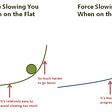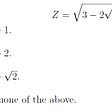# Sorting Algorithms

• What is a stable sorting algorithm? Algorithms that maintain the relative order of records with equal keys (values) are stable.
• What is an in-place sorting algorithm? Algorithms in which the sorted items occupy the same storage as the original ones are in place. This simply means there is no auxiliary space requirement for storing the sorted items
• The bubble sort is the simplest sorting algorithm. Due to its simplicity, bubble sort is often used to introduce the concept of a sorting algorithm
• This algorithm works by swapping the adjacent elements time after time if they violate the sorted order.
• The average and worst-case time complexity of bubble sort is O(n*n), where the worst-case occurs when the input is reversely sorted
• Bubble sort can be optimized by stopping the algorithm if no swap occurred. This optimized version can be used to detect whether the input is already sorted, that too in a linear time!
• Bubble sort is a stable and in-place sorting algorithm
• The selection sort works by finding the minimum element (considering ascending order) time after time from the unsorted part and places it at the beginning of the unsorted subarray.
• This algorithm maintains two subarrays in a given array. In first, the subarray is sorted. The second contains the remaining subarray.
• At each iteration of the selection sort, the unsorted part's minimum element is picked and moved to the sorted subarray.
• The worst and best case time complexity of selection sort is O(n*n), this happens because no matter what the input is, the algorithm will scan the array n times (n is the size) each time finding the minimum element which in turn takes n iterations
• Selection sort is an in-place algorithm. The default implementation of this algorithm is not stable. However, it can be made stable. For a more detailed explanation, click here
• An interesting thing about selection sort is it never makes more than n swaps (n is the size) and therefore can be useful when swapping operation is costly. Besides, it acts as a base for a more powerful sorting algorithm, the heap sort
• Insertion sort is a sorting algorithm that moves an element to its right position at each iteration. The array is virtually broken into sorted and unsorted parts. Values from the unsorted subarray are picked and moved to the correct spot in the sorted subarray.
• The worst-case time complexity of insertion sort is O(n*n), which occurs when the array is reversely sorted. The best-case (O(n)) occurs when the array is already sorted.
• Insertion sort is useful in situations where the size of the array is small or where the input is almost sorted. Besides, it also acts as a base for a more powerful sorting algorithm, the shell sort
• This is a stable and in-place sorting algorithm

--

--

--

Love podcasts or audiobooks? Learn on the go with our new app.

## Recommended from Medium## Nothing is continuous## Human Right That Could Result In Cheeps## Random Variables and Distributions.## Climbing@250w vs Flat@250w: Is there a difference?## A Cambridge University Interview Question. Can You Solve It?## Full Stack Group Project?! Here is how we did it.